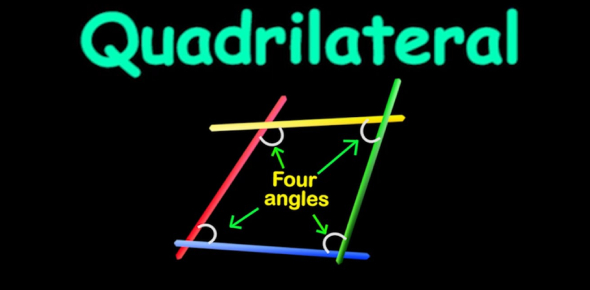Quadrilateral Quiz: Can You Pass This Test?

15 Questions | Total Attempts: 865SettingsQuadrilateral quiz: can you pass this test? How good are you when it comes to solving quadrilateral equations and angles? This quiz is specifically designed for those who have a hard time with these math problems and are looking for some revision material. Do give it a try and remember to keep an eye out for other quizzes just like it to polish up your skills. All the best!

• 1.
A diagonal of the parallelogram divides it into:
• A.

Two triangles

• B.

Two congruent triangles

• C.

Two triangles of equal area

• D.

All of the above

• 2.
• A.

40o

• B.

45o

• C.

50o

• D.

60o

• 3.
• A.

80o

• B.

100o

• C.

120o

• D.

160o

• 4.
In a quadrilateral three angles are in a ratio 3 : 3: 1 and one  of the angles is 80o, then other angles are:
• A.
• B.
• C.
• D.
• 5.
In a quadrilateral ABCD,diagonals bisect each other at right angles. Also, AB = BC = AD = 6cm, then the length of CD is:
• A.

3 cm

• B.

6 cm

• C.
• D.

12 cm

• 6.
Two consecutive angles are in the ratio 1 : 3, then the smaller angle is:
• A.

45°

• B.

50°

• C.

60°

• D.

90°

• 7.
A quadrilateral whose diagonals are equal and bisect each other at right angles is a:
• A.

Rhombus

• B.

Square

• C.

Trapezium

• D.

Rectangle

• 8.
• A.

0o

• B.

90o

• C.

120o

• D.

180o

• 9.
The Sum of all interior angles of a quadrilateral is:
• A.

90o

• B.

180o

• C.

360o

• D.

540o

• 10.
In a trapezium ABCD with AB = 20 cm, CD = 10 cm, AB || CD, P, and Q are midpoints of AC and BD then the length of PQ is:
• A.

10 cm

• B.

5 cm

• C.

2 cm

• D.

None

• 11.
If M is the midpoint of hypotenuse PR of a right-angled triangle PQR right-angled at Q then,
• A.

MP = MR

• B.

MQ = MR

• C.

MQ = MP

• D.

All of these

• 12.
The figure obtained by joining the midpoints of the sides of a rhombus, taken in order, yes:
• A.

A rhombus

• B.

A rectangle

• C.

A square

• D.

Any parallelogram

• 13.
• A.

A square

• B.

A rectangle

• C.

A rhombus

• D.

A parallelogram

• 14.
The figure formed by joining the midpoints of the sides of a quadrilateral ABCD, taken in order, is a square only if,
• A.

ABCD is a rhombus

• B.

Diagonals of ABCD are equal

• C.

Diagonals of ABCD are equal and perpendicular

• D.

Diagonals of ABCD are perpendicular

• 15.
Two angles of a quadrilateral are 60o and 70o and other two angles are in the ratio of 8:15 then the remaining two angles are:
• A.
• B.
• C.
• D.
Related TopicsBack to top